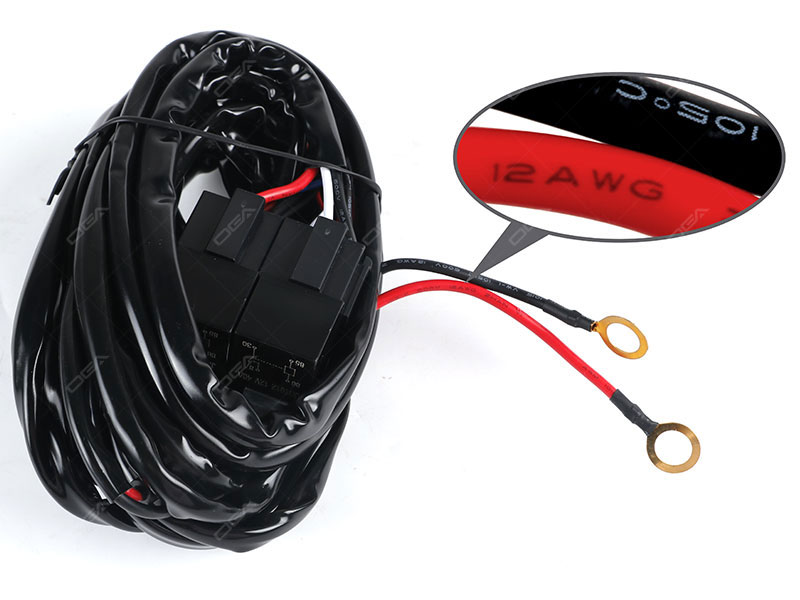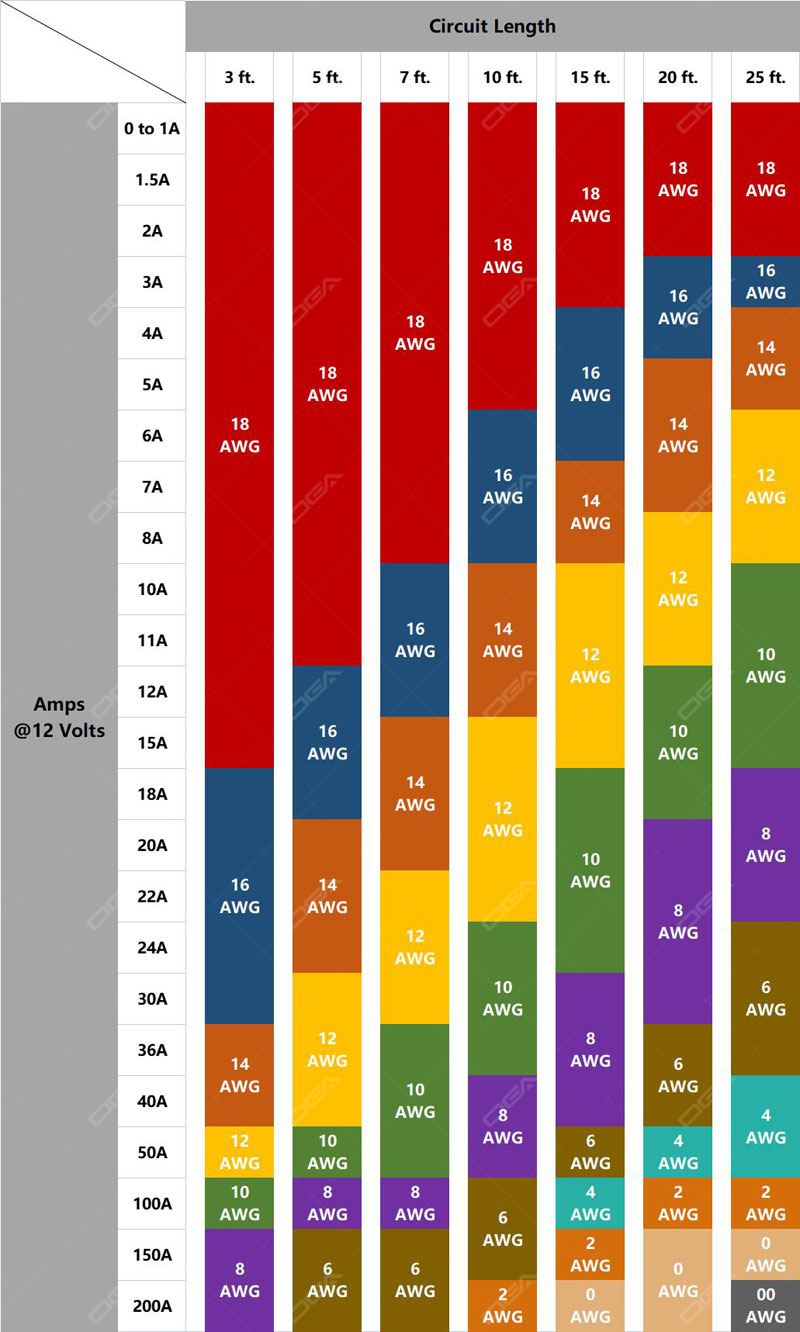# How to Select the Right Wire for Automotive Applications

Date：2022-05-23  Source：OGAWire harness for OGA LED's 54 series LED light bars

Selecting the right wiring for automotive applications and maintenance of electrical systems is an integral part of keeping the vehicle's electrical systems operating as designed. There are many types of automotive wire, and understanding which types are best suited for the application can be confusing. For this reason, today we will focus on wire typically used in automotive applications, how to choose the right size wire, run the right size wire for the load and don't run too small wire.

It's a little bit complex of the topic, we want to cover a few background scientific factors that go into this in simple terms.

First and foremost is voltage, voltage is a measure of electrical potential. In slightly more complex terms, voltage is a quantitative expression of the difference in charge between two points in the electrical field.

The next thing needs to understand is voltage drop. A voltage drop is the loss of voltage over a circuit, this can come in the form of a load, for example, of a light bulb. They're using voltage so they are dropping the voltage, that's an obvious one. But wire itself can be a voltage drop, drop voltage also happen in a wire.

And the third thing to understand here is amperage, which is a current, the actual load device, and how much amperage it is using or wattage. Depending on the given information, amperage can be figured out based on wattage and voltage, we'll get to that a little later.

And lastly, what is a wire gauge?
A wire gauge is a measurement of the diameter of the wire. This size determines the amount of current the wire can safely handle, and it also tells the weight and electrical resistance of the wire. The wire is typically labeled or referred to with a gauge number, followed by "AWG." Perhaps you've seen this abbreviation before, it stands for "American Wire Gauge." The most common wire gauge sizes seen in automotive applications include 8, 10, 12, 14, 16 and 18 gauge.

 AWG Number Dia.(mm) Ampacity mΩ/ft 0 8.251 125A 0.1 4 5.189 70A 0.25 7 3.665 / 0.50 10 2.588 30A 1 20 0.812 5A 10 40 0.080 / 1000

From the table above we can tell that the higher number corresponds to a smaller wire. A wire gauge of 0 has a diameter size of about 8.251 millimeters, whereas a wire with a gauge of 40 has a diameter of 0.080 millimeters. So as the wire gauge increases in number, the diameter or the size of the wire decreases, these two are inversely related.

A wire gauge of 4 can handle a current of 70 amps, whereas a wire with a gauge of 20 can handle a current of 5 amps. So as the gauge number increases, the amount of current that it can handle decreases.

The other thing we need to talk about is the resistance per unit length, the resistance per unit length of a wire with a size of 7 is 0.50 milliohm per foot, whereas the resistance per unit length for a wire with a size of 10 is 1 milliohm per foot, so as the wire gauge increases in value, the resistance per unit of length increases as well.

So these are the things to keep in mind, as the wire gauge increases, the diameter size decreases, the ampacity (the amount of current that the wire can handle) decreases, but the resistance increases.

How to Select the Right Wire Gauge
So now we have broken down this concept into some simpler terms, and we mentioned a couple of the items and the important parts that are involved in this process. To find the right wire gauge, we need to know the current voltage drop, and that's the amount of voltage lost over the length of the wire.

As we mentioned before, a voltage drop happens in a wire because the wire has resistance. So there's a voltage drop that resistance is consuming voltage as the voltage is traveling through the wire. That means the longer the wire is, the greater resistance of that wire, and the more voltage drop.

Voltage drop changes as a function of the resistance of the wire, and should be less than 3% if possible. If the drop is greater than 3%, the efficiency of the equipment in the circuit is severely decreased and the life of the equipment will be decreased. As circuit lengths get longer, electrical resistance builds up until it forces the voltage down below a usable level. And this is the point of this article, we should be able to foresee that and use a wire of a larger gauge number that's going to restore the voltage to its intended level.

Let us give an example, when we're wiring, if we need to supply an off-road auxiliary light with good strong power, a 20 gauge wire is probably not a good choice, because we need to go from the auxiliary light switch to the driver side auxiliary light, to the passenger side auxiliary light, depending on how we have it wired, that could be a decent distance. On a big enough vehicle that could be 10 or 15 feet, until it gets to that other light, we might well lose upwards of a volt or a volt and a half from our switch to our load device, that means if we're only getting eleven or ten and a half volts to an auxiliary light, that's going to dim the light.

Light needs a certain amount of amperage, in another word, the voltage has to supply enough amperage to the light. As amperage flows from the battery down the wire to the light, it needs to have a large enough wire to supply enough amperage to that light without resistance in that wire affecting it, so that the light can function properly.

So how is voltage drop calculated? Ohm's Law states that current through a conductor between two points is directly proportional to the potential difference across the two points. That is a very long way of saying, Voltage Drop equals Current in amperes multiples by Resistance in ohms. According to the calculated voltage drop, we should be able to find the right wire size.

But there are ways to determine how big of a wire we're going to run on an item, and that is to check how much power that item is going to draw. We can refer to the manual, or refer to the instructions of a device, and see if it lists the amperage that they're expecting, the amperage they want it fused at, or the wattage that item is going to pull, to determine the wire size that way. If they only provide us with a wattage, we can determine a bridge based on wattage and voltage.

For example, with an auxiliary light, maybe we've got a 60 watt premium work light from OGA LED, divide that by the 12-volt power that we're supplying to it, and we've got an amperage draw of 5 amps. But normally we would have a pair of thses 7" heavy duty LED lights, that's going to pull a little over 10 amps on those two lights. That doesn't sound like a lot of power, but it's a constant flow of power, also it can be over a decent distance to get there. So when it comes to an auxiliary light circuit, we aim for a 16 gauge where a minimum of an 18 gauge wire on that circuit.

If it's something like a taillight, a turn signal, or for purely decorative purposes like OGA LED's Jeep Grill daytime running light, that's not a heavy wattage circuit, it's not pulling a lot of power and it's not under as constant of a load as say an auxiliary light is or a LED light bar, that we would go with like an 18 gauge or a 20 gauge depending on the distance for that circuit.

We're going to go ahead and throw a picture down there to a chart that breaks down our recommended wire sizes for different circuits in a vehicle.LAST NEXTTechnical Support: Magic Lamp